Successfully reported this slideshow.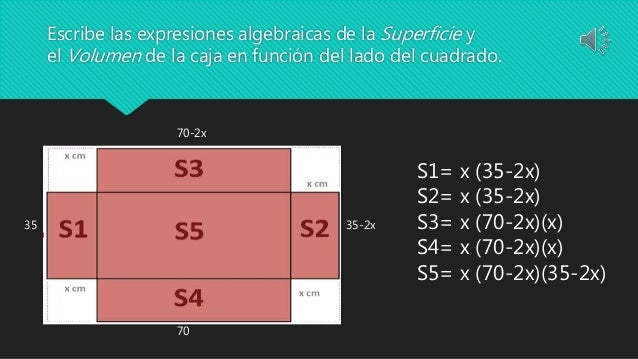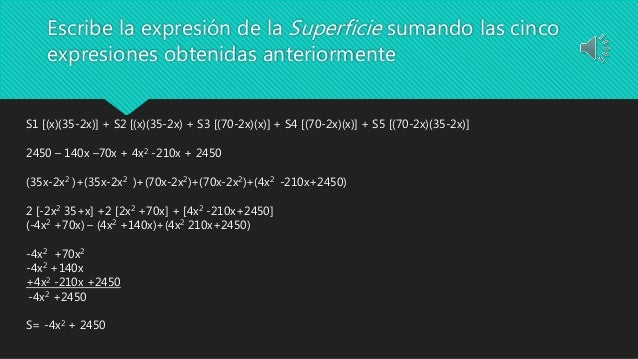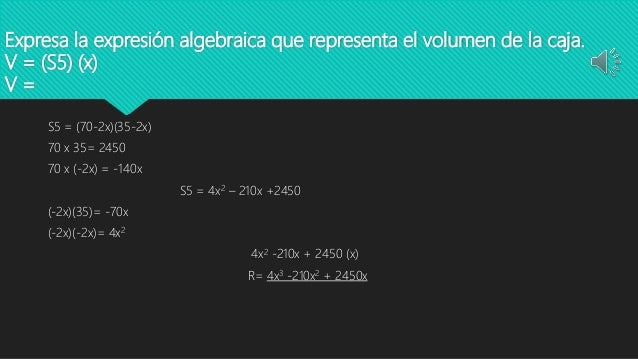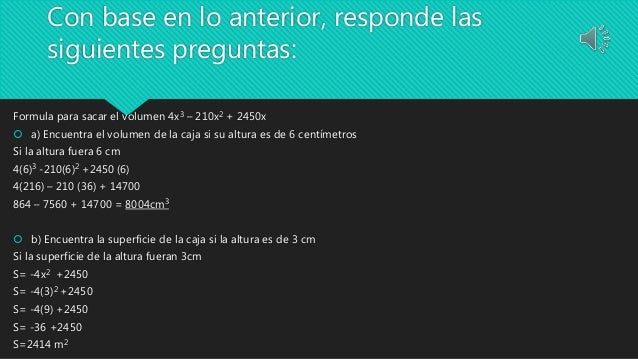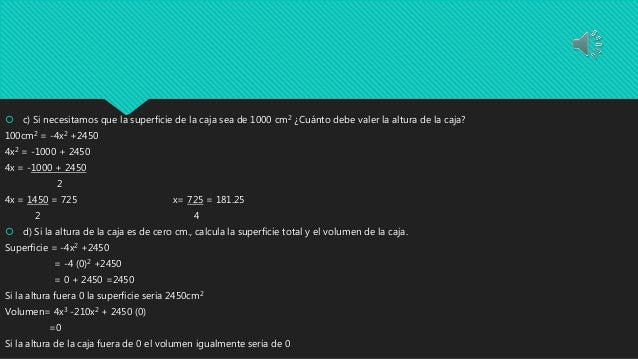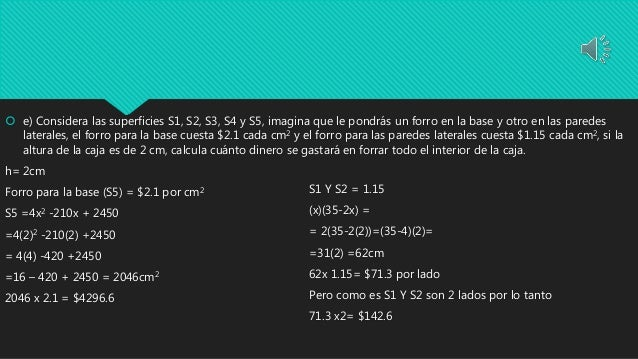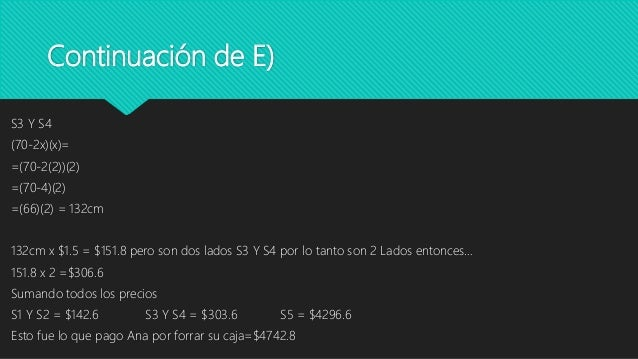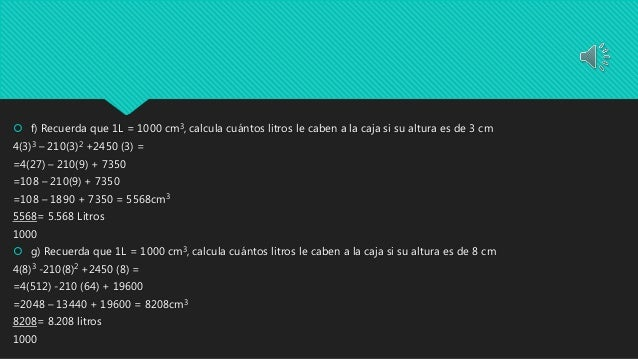Upcoming SlideShare
×
• Full Name
Comment goes here.

Are you sure you want to Yes No• Be the first to comment

### Ocañas gongora orlandoisrael_m11s4_proyecto_reutilizando

1. 1. Proyecto integrador Reutilizando Orlando Israel Ocañas Góngora Modulo 11
2. 2. Escribe las expresiones algebraicas de la Superficie y el Volumen de la caja en función del lado del cuadrado. 70-2x 35-2x 70 35 S1= x (35-2x) S2= x (35-2x) S3= x (70-2x)(x) S4= x (70-2x)(x) S5= x (70-2x)(35-2x)
3. 3. Escribe la expresión de la Superficie sumando las cinco expresiones obtenidas anteriormente S1 [(x)(35-2x)] + S2 [(x)(35-2x) + S3 [(70-2x)(x)] + S4 [(70-2x)(x)] + S5 [(70-2x)(35-2x)] 2450 – 140x –70x + 4x2 -210x + 2450 (35x-2x2 )+(35x-2x2 )+(70x-2x2)+(70x-2x2)+(4x2 -210x+2450) 2 [-2x2 35+x] +2 [2x2 +70x] + [4x2 -210x+2450] (-4x2 +70x) – (4x2 +140x)+(4x2 210x+2450) -4x2 +70x2 -4x2 +140x +4x2 -210x +2450 -4x2 +2450 S= -4x2 + 2450
4. 4. Expresa la expresión algebraica que representa el volumen de la caja. V = (S5) (x) V = S5 = (70-2x)(35-2x) 70 x 35= 2450 70 x (-2x) = -140x S5 = 4x2 – 210x +2450 (-2x)(35)= -70x (-2x)(-2x)= 4x2 4x2 -210x + 2450 (x) R= 4x3 -210x2 + 2450x
5. 5. Con base en lo anterior, responde las siguientes preguntas: Formula para sacar el volumen 4x3 – 210x2 + 2450x  a) Encuentra el volumen de la caja si su altura es de 6 centímetros Si la altura fuera 6 cm 4(6)3 -210(6)2 +2450 (6) 4(216) – 210 (36) + 14700 864 – 7560 + 14700 = 8004cm3  b) Encuentra la superficie de la caja si la altura es de 3 cm Si la superficie de la altura fueran 3cm S= -4x2 +2450 S= -4(3)2 +2450 S= -4(9) +2450 S= -36 +2450 S=2414 m2
6. 6.  c) Si necesitamos que la superficie de la caja sea de 1000 cm2 ¿Cuánto debe valer la altura de la caja? 100cm2 = -4x2 +2450 4x2 = -1000 + 2450 4x = -1000 + 2450 2 4x = 1450 = 725 x= 725 = 181.25 2 4  d) Si la altura de la caja es de cero cm., calcula la superficie total y el volumen de la caja. Superficie = -4x2 +2450 = -4 (0)2 +2450 = 0 + 2450 =2450 Si la altura fuera 0 la superficie seria 2450cm2 Volumen= 4x3 -210x2 + 2450 (0) =0 Si la altura de la caja fuera de 0 el volumen igualmente seria de 0
7. 7.  e) Considera las superficies S1, S2, S3, S4 y S5, imagina que le pondrás un forro en la base y otro en las paredes laterales, el forro para la base cuesta \$2.1 cada cm2 y el forro para las paredes laterales cuesta \$1.15 cada cm2, si la altura de la caja es de 2 cm, calcula cuánto dinero se gastará en forrar todo el interior de la caja. h= 2cm Forro para la base (S5) = \$2.1 por cm2 S5 =4x2 -210x + 2450 =4(2)2 -210(2) +2450 = 4(4) -420 +2450 =16 – 420 + 2450 = 2046cm2 2046 x 2.1 = \$4296.6 S1 Y S2 = 1.15 (x)(35-2x) = = 2(35-2(2))=(35-4)(2)= =31(2) =62cm 62x 1.15= \$71.3 por lado Pero como es S1 Y S2 son 2 lados por lo tanto 71.3 x2= \$142.6
8. 8. Continuación de E) S3 Y S4 (70-2x)(x)= =(70-2(2))(2) =(70-4)(2) =(66)(2) = 132cm 132cm x \$1.5 = \$151.8 pero son dos lados S3 Y S4 por lo tanto son 2 Lados entonces… 151.8 x 2 =\$306.6 Sumando todos los precios S1 Y S2 = \$142.6 S3 Y S4 = \$303.6 S5 = \$4296.6 Esto fue lo que pago Ana por forrar su caja=\$4742.8
9. 9.  f) Recuerda que 1L = 1000 cm3, calcula cuántos litros le caben a la caja si su altura es de 3 cm 4(3)3 – 210(3)2 +2450 (3) = =4(27) – 210(9) + 7350 =108 – 210(9) + 7350 =108 – 1890 + 7350 = 5568cm3 5568= 5.568 Litros 1000  g) Recuerda que 1L = 1000 cm3, calcula cuántos litros le caben a la caja si su altura es de 8 cm 4(8)3 -210(8)2 +2450 (8) = =4(512) -210 (64) + 19600 =2048 – 13440 + 19600 = 8208cm3 8208= 8.208 litros 1000

### Be the first to comment

•May. 9, 2017

Total views

147

On Slideshare

0

From embeds

0

Number of embeds

0

2

Shares

0